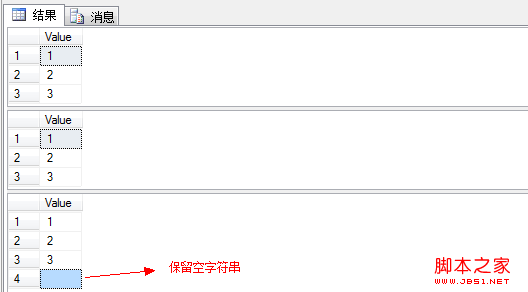# SQL里面用自定义Split()完成个性化需求

更新时间：2013年02月21日 15:06:14   作者：我要评论

SET ANSI_NULLS ON
GO
SET QUOTED_IDENTIFIER ON
GO
CREATE function [dbo].[SplitString]
(
@Input nvarchar(max),
@Separator nvarchar(max)=',',
@RemoveEmptyEntries bit=1
)
returns @TABLE table
(
[Id] int identity(1,1),
[Value] nvarchar(max)
)
as
begin
declare @Index int, @Entry nvarchar(max)
set @Index = charindex(@Separator,@Input)
while (@Index>0)
begin
set @Entry=ltrim(rtrim(substring(@Input, 1, @Index-1)))
if (@RemoveEmptyEntries=0) or (@RemoveEmptyEntries=1 and @Entry<>'')
begin
insert into @TABLE([Value]) Values(@Entry)
end
set @Input = substring(@Input, @Index+datalength(@Separator)/2, len(@Input))
set @Index = charindex(@Separator, @Input)
end
set @Entry=ltrim(rtrim(@Input))
if (@RemoveEmptyEntries=0) or (@RemoveEmptyEntries=1 and @Entry<>'')
begin
insert into @TABLE([Value]) Values(@Entry)
end
return
end

declare @str1 varchar(max), @str2 varchar(max), @str3 varchar(max)
set @str1 = '1,2,3'
set @str2 = '1###2###3'
set @str3 = '1###2###3###'
select [Value] from [dbo].[SplitString](@str1, ',', 1)
select [Value] from [dbo].[SplitString](@str2, '###', 1)
select [Value] from [dbo].[SplitString](@str3, '###', 0)